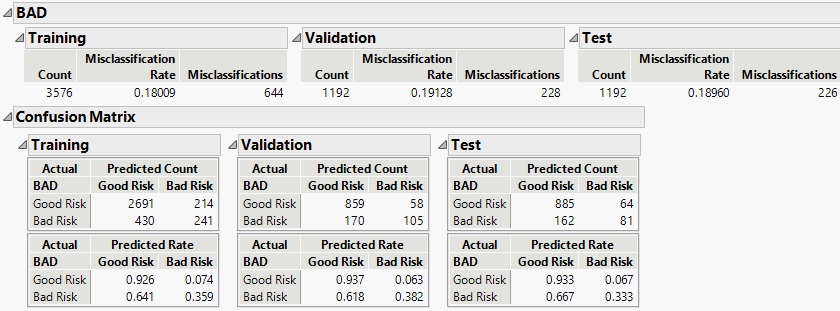Predictive and Specialized Modeling > Naive Bayes > Additional Example of Naive Bayes
Publication date: 11/10/2021

#Additional Example of Naive Bayes

You have historical financial data for 5,960 customers who applied for home equity loans. Each customer was classified as being a Good Risk or Bad Risk. There is missing data on most of the predictors. You want to construct a model to use in classifying the credit risk of future customers.

1. Select Help > Sample Data Library and open Equity.jmp.

2. Select Analyze > Predictive Modeling > Naive Bayes.

3. Select BAD and click Y, Response.

One of the potential predictors, DEBTINC, has many missing values that might be informative. However, naive Bayes is not prepared to handle large number of missing values well, so you do not include DEBTINC in your model.

4. Select LOAN through CLNO and click X, Factor.

5. Select Validation and click Validation.

6. Click OK.

Figure 8.8 Naive Bayes Report for BADThe Training, Validation, and Test sets show misclassification rates between 18% and 19%. The confusion matrices for all of the sets suggest that the largest source of misclassification is the classification of Bad Risk customers as Good Risk customers.

You are interested in the probabilities that customers with certain financial background values are classified as High Risk.

7. Click the Naive Bayes red triangle and select Save Probability Formulas.

Three sets of columns are added to the data table.

The three Naive Score columns contain naive score formulas for Good Risk, Bad Risk, and the sum of both.

The two Naive Prob columns contain probability formulas for Good Risk and Bad Risk.

The Naive Predicted Formula Bad column contains a formula that assigns an observation to the class for which the observation has the highest naive probability.

Use these formulas to score new customers. For more information about the formula columns, see Saved Probability Formulas.